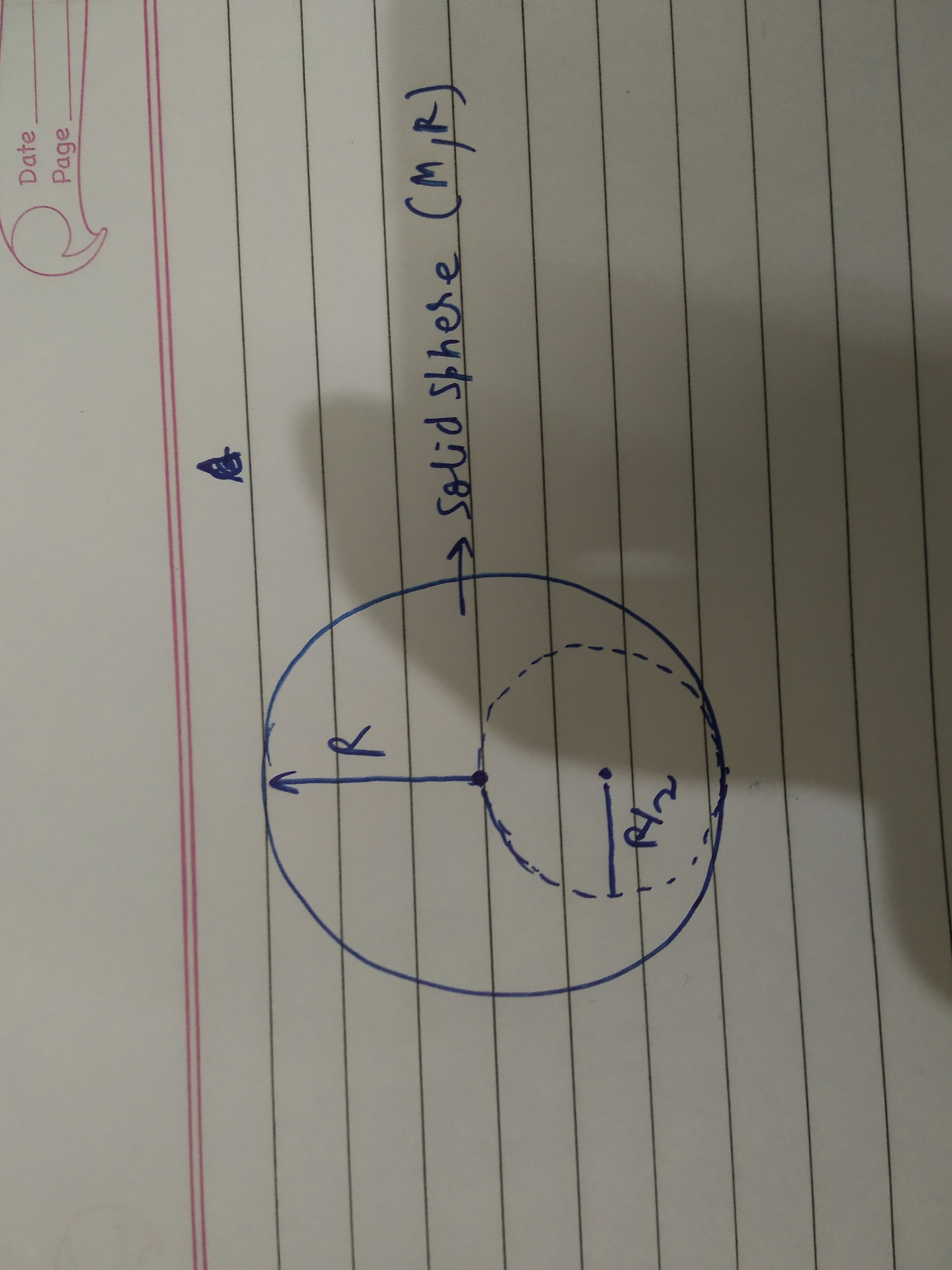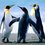# Tough Gravitation problem

A uniform solid sphere has a Mass M and radius R. From it , a solid sphere of radius R/2 is removed and taken to infinity . Can anyone help to calculate the work done by external agent to achieve this ?( Can integration over three dimensions be avoidef here ?) ( I am not getting how to solve as the gravitatonal field will be not uniform inside the cavity sphere )Note by Kudo Shinichi
1 year, 10 months ago

This discussion board is a place to discuss our Daily Challenges and the math and science related to those challenges. Explanations are more than just a solution — they should explain the steps and thinking strategies that you used to obtain the solution. Comments should further the discussion of math and science.

When posting on Brilliant:

• Use the emojis to react to an explanation, whether you're congratulating a job well done , or just really confused .
• Ask specific questions about the challenge or the steps in somebody's explanation. Well-posed questions can add a lot to the discussion, but posting "I don't understand!" doesn't help anyone.
• Try to contribute something new to the discussion, whether it is an extension, generalization or other idea related to the challenge.
• Stay on topic — we're all here to learn more about math and science, not to hear about your favorite get-rich-quick scheme or current world events.

MarkdownAppears as
*italics* or _italics_ italics
**bold** or __bold__ bold
- bulleted- list
• bulleted
• list
1. numbered2. list
1. numbered
2. list
Note: you must add a full line of space before and after lists for them to show up correctly
paragraph 1paragraph 2

paragraph 1

paragraph 2

[example link](https://brilliant.org)example link
> This is a quote
This is a quote
    # I indented these lines
# 4 spaces, and now they show
# up as a code block.

print "hello world"
# I indented these lines
# 4 spaces, and now they show
# up as a code block.

print "hello world"
MathAppears as
Remember to wrap math in $$ ... $$ or $ ... $ to ensure proper formatting.
2 \times 3 $2 \times 3$
2^{34} $2^{34}$
a_{i-1} $a_{i-1}$
\frac{2}{3} $\frac{2}{3}$
\sqrt{2} $\sqrt{2}$
\sum_{i=1}^3 $\sum_{i=1}^3$
\sin \theta $\sin \theta$
\boxed{123} $\boxed{123}$

## Comments

Sort by:

Top Newest

Integrating numerically, I get very nearly $\frac{G M^2}{8R}$.

  1 2 3 4 5 6 7 8 9 10 11 12 13 14 15 16 17 18 19 20 21 22 23 24 25 26 27 28 29 30 31 32 33 34 35 36 37 38 39 40 41 42 43 44 45 46 47 48 49 50 51 52 53 54 55 56 57 58 59 60 61 62 63 64 65 66 67 68 69 70 71 72 73 74 75 76 77 78 79 80 81 82 83 84 85 86 import math N = 500 # simulation resolution ########################################## # Constants M = 2.7 # Full sphere mass R = 1.3 # Full sphere radius G = 11.1 # Grav constant V = (4.0/3.0)*math.pi*(R**3.0) # Full sphere volume rho = M/V # density Rs = R/2.0 # small sphere radius Vs = (4.0/3.0)*math.pi*(Rs**3.0) # Small sphere volume Ms = Vs * rho # Small sphere mass U_ref = G*(M**2.0)/(8.0*R) # Reference value of work done - for comparison ########################################## # Infinitesimals for integration dr = R/N dtheta = 2.0*math.pi/N dphi = math.pi/N ########################################## r = 0.0 U = 0.0 count = 0 while r <= R: # Integrate over interior of large sphere theta = 0.0 while theta <= 2.0*math.pi: phi = 0.0 while phi <= math.pi: x = r*math.cos(theta)*math.sin(phi) # xyz coordinates y = r*math.sin(theta)*math.sin(phi) z = r*math.cos(phi) # (x-Rs)**2.0 + y**2.0 + z**2.0 = Rs**2.0 if (x-Rs)**2.0 + y**2.0 + z**2.0 > Rs**2.0: # For points within large sphere.... # .....and outside small sphere Dx = x - Rs # vector from infinitesimal mass to center of small sphere Dy = y - 0.0 Dz = z - 0.0 D = math.sqrt(Dx**2.0 + Dy**2.0 + Dz**2.0) # distance from mass dm to small sphere center dV = (r**2.0)*math.sin(phi) * dr * dtheta * dphi # infinitesimal volume dm = rho * dV # infinitesimal mass dU = G*Ms*dm/D # infinitesimal potential energy contribution U = U + dU # update total potential energy value phi = phi + dphi count = count + 1 if count%10**6 == 0: print count/10**6 theta = theta + dtheta r = r + dr ########################################## print "" print "" print U/U_ref 

- 1 year, 9 months ago

Log in to reply

Very nice , thx a lot Sir

- 1 year, 9 months ago

Log in to reply

@Steven Chase Sir have a look at this interesting problem .

- 1 year, 10 months ago

Log in to reply

I think I would use a triple integral and a computer to solve this

- 1 year, 10 months ago

Log in to reply

Sir , can u give some intial steps to proceed this problem ?

- 1 year, 10 months ago

Log in to reply

Do you know what the answer is supposed to be? I would like to check my numerical result, once derived.

- 1 year, 10 months ago

Log in to reply

Yeah sure , Sir. Very sry for late reply

- 1 year, 9 months ago

Log in to reply

What is the expected result?

- 1 year, 9 months ago

Log in to reply

Sir i think it should be close to GM^2/8R

- 1 year, 9 months ago

Log in to reply

×

Problem Loading...

Note Loading...

Set Loading...